# BODY COMPOSITION BMI What is Body Composition In

• Slides: 6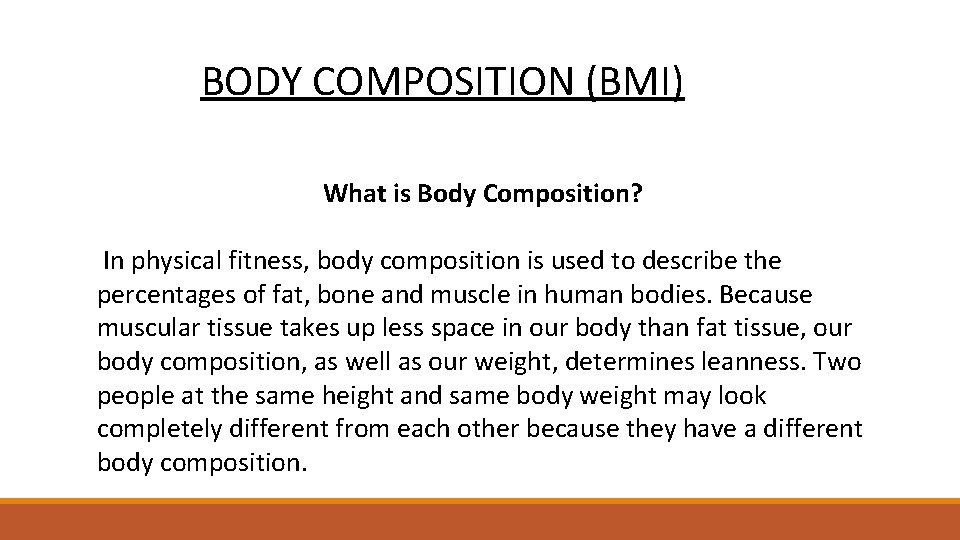BODY COMPOSITION (BMI) What is Body Composition? In physical fitness, body composition is used to describe the percentages of fat, bone and muscle in human bodies. Because muscular tissue takes up less space in our body than fat tissue, our body composition, as well as our weight, determines leanness. Two people at the same height and same body weight may look completely different from each other because they have a different body composition.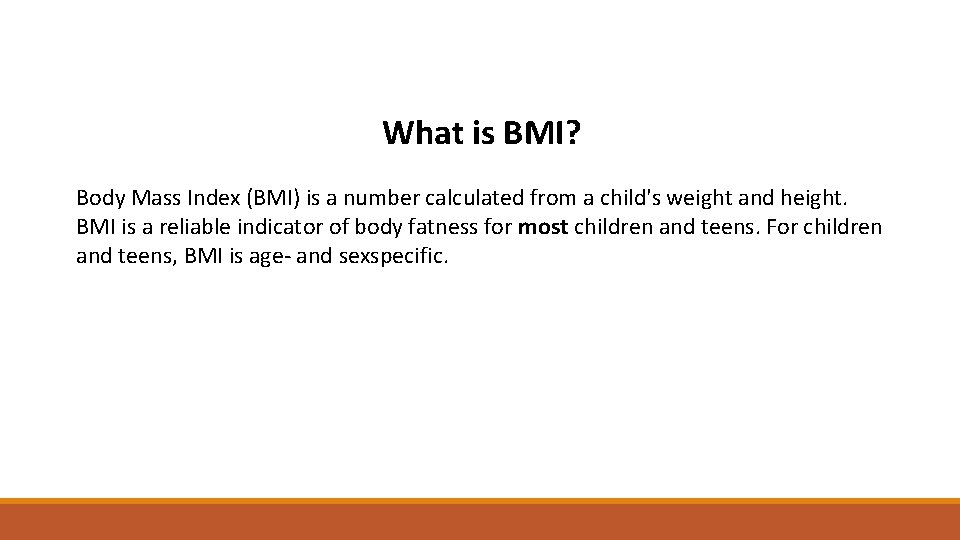What is BMI? Body Mass Index (BMI) is a number calculated from a child's weight and height. BMI is a reliable indicator of body fatness for most children and teens. For children and teens, BMI is age- and sexspecific.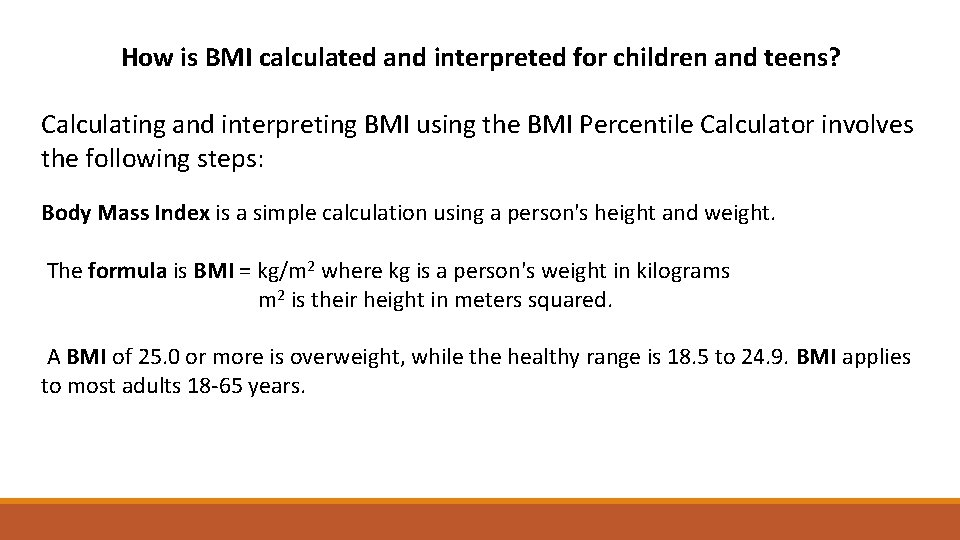How is BMI calculated and interpreted for children and teens? Calculating and interpreting BMI using the BMI Percentile Calculator involves the following steps: Body Mass Index is a simple calculation using a person's height and weight. The formula is BMI = kg/m 2 where kg is a person's weight in kilograms m 2 is their height in meters squared. A BMI of 25. 0 or more is overweight, while the healthy range is 18. 5 to 24. 9. BMI applies to most adults 18 -65 years.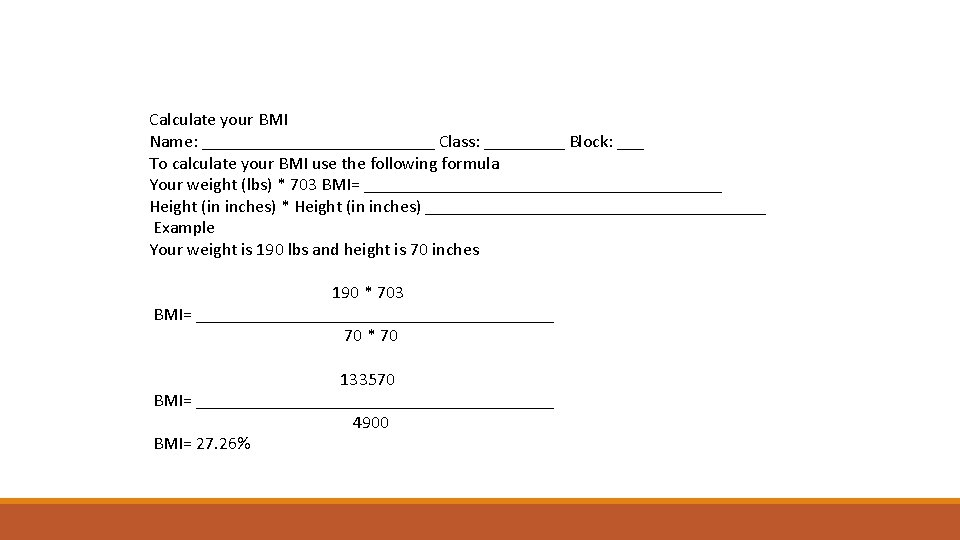Calculate your BMI Name: _____________ Class: _____ Block: ___ To calculate your BMI use the following formula Your weight (lbs) * 703 BMI= ____________________ Height (in inches) * Height (in inches) ___________________ Example Your weight is 190 lbs and height is 70 inches 190 * 703 BMI= ____________________ 70 * 70 133570 BMI= ____________________ 4900 BMI= 27. 26%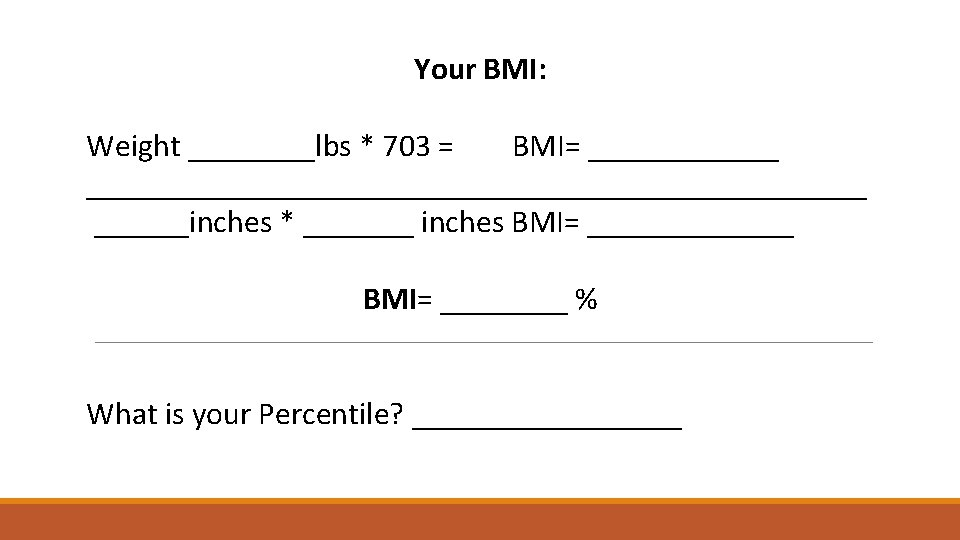Your BMI: Weight ____lbs * 703 = BMI= _______________________________inches * _______ inches BMI= _______ BMI= ____ % What is your Percentile? _________BMI: Male / Female Chart